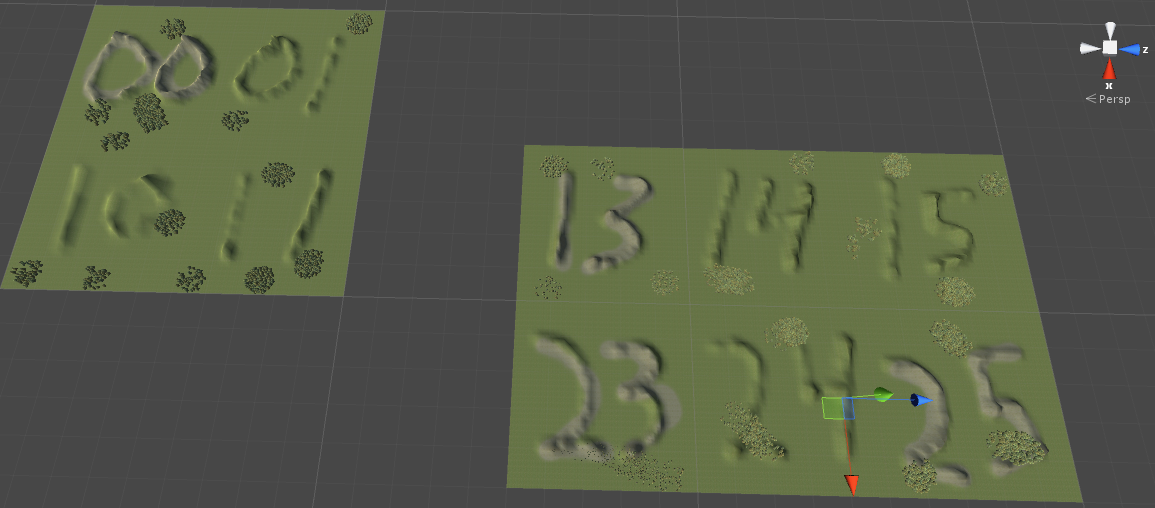# Simple Multiplayer Terrain Manager for Unity

Here is some code I wrote to load terrain only where the player is. We don’t load the entire map, just the current and neighboring areas.

We will store the terrain data in a dictionary that uses an X and Z coordinate system as a key (Unity uses Z for east-west).

 0, 0 0, 1 0, 2 1, 0 1, 1 1, 2 2, 0 2, 1 2, 2

Pictured is the final result with one player at 0, 0 and another at 2, 4. Note this view is from the server where all terrain in use by clients must be loaded. Clients will only load what is needed for their view.Since we are storing terrain in a dictionary, our TerrainKey will be important to understand. Here is how we assign a TerrainKey:

```const int xMax = 3; // How many tiles our north-south is
const int zMax = 6; // How many tiles east-west is
const float terrainSize = 500.0f; // Size of each terrain tile

// Unity uses z instead of y for east-west
public TerrainKey(int x, int z)
{
this.x = x;
this.z = z;
}

// Get key from position
public TerrainKey(Vector3 pos)
{
x = Mathf.FloorToInt(pos.x / terrainSize);
z = Mathf.FloorToInt(pos.z / terrainSize);
}

// Might be outside range
public bool isValid()
{
if (x < 0 || x >= xMax)
return false;
if (z < 0 || z >= zMax)
return false;
return true;
}

public override string ToString()
{
return string.Format("Terrain{0}_{1}", x, z);
}
```

Every second we will have our players report their position. We will also want to calculate the neighboring terrain. Note we are marking each grid spot when it is needed by saving a time value. The TerrainManager uses this to know what terrain to load or remove.

```// Called by player objects
public static void reportLocation(Vector3 pos)
{
if (instance == null)
return;
TerrainKey key = new TerrainKey(pos);
if (!key.isValid())
{
Debug.Log("Player outside terrain area");
return;
}

// Mark neighbors as being needed
TerrainKey[] neighbors = key.getNeighbors();
for (int i = 0; i < neighbors.Length; i++)
{
key = neighbors[i];
}
}
```

Here is the function the TerrainManager is calling every second to check if it needs to load or remove terrain. Note we load the terrain resource asynchronously.

```// As terrain is flagged as needed or not needed this routine will load or destroy terrain
IEnumerator manageDictionary()
{
while (true)
{
foreach (var pair in terrainDictionary)
{
{
if (pair.Value.gameObject == null)
continue;

// Don't need terrain so remove
Destroy(pair.Value.gameObject);
}
else
{
if (pair.Value.gameObject != null)
continue;

{
}
}
}

// Check every second
yield return new WaitForSeconds(1.0f);
}
}

// Loads terrain from resource and sets to correct position
{
yield return null; // Starts again when LoadAsync is done

TerrainData t = request.asset as TerrainData;
pair.Value.gameObject = Terrain.CreateTerrainGameObject(t);
pair.Value.gameObject.transform.position = pair.Key.getPos();